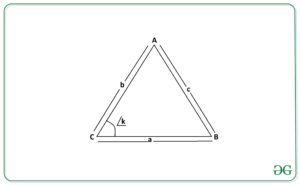Open In App

# Area of Triangle using Side-Angle-Side (length of two sides and the included angle)

Given two integers A, B representing the length of two sides of a triangle and an integer K representing the angle between them in radian, the task is to calculate the area of the triangle from the given information.
Examples:

Input: a = 9, b = 12, K = 2
Output: 49.1
Explanation:
Area of triangle = 1 / 2 * (9 * 12 * Sin 2) = 35.12
Input: A = 2, B = 4, K = 1
Output: 3.37

Approach:
Consider the following triangle ABC with sides A, B, C, and an angle K between sides A and BThen, the area of the triangle can be calculated using the Side-Angle-Side formula:Below is the implementation of the above approach:

## C++

 // C++ program to calculate// the area of a triangle when// the length of two adjacent// sides and the angle between// them is provided#include using namespace std; float Area_of_Triangle(int a, int b, int k){    float area = (float)((1 / 2.0) *                          a * b * (sin(k)));     return area;} // Driver Codeint main(){    int a = 9;    int b = 12;    int k = 2;     // Function Call    float ans = Area_of_Triangle(a, b, k);     // Print the final answer    cout << ans << endl;} // This code is contributed by Ritik Bansal

## Java

 // Java program to calculate// the area of a triangle when// the length of two adjacent// sides and the angle between// them is providedclass GFG{ // Function to return the area of// triangle using Side-Angle-Side// formulastatic float Area_of_Triangle(int a, int b,                                     int k){    float area = (float)((1 / 2.0) *                      a * b * Math.sin(k));     return area;} // Driver Codepublic static void main(String[] args){    int a = 9;    int b = 12;    int k = 2;     // Function Call    float ans = Area_of_Triangle(a, b, k);     // Print the final answer    System.out.printf("%.1f",ans);}} // This code is contributed by sapnasingh4991

## Python3

 # Python3 program to calculate# the area of a triangle when# the length of two adjacent# sides and the angle between# them is provided import math # Function to return the area of# triangle using Side-Angle-Side# formuladef Area_of_Triangle(a, b, k):     area =(1 / 2) * a * b * math.sin(k)     return area # Driver Codea = 9b = 12k = 2 # Function Callans = Area_of_Triangle(a, b, k) # Print the final answerprint(round(ans, 2))

## C#

 // C# program to calculate// the area of a triangle when// the length of two adjacent// sides and the angle between// them is providedusing System;class GFG{ // Function to return the area of// triangle using Side-Angle-Side// formulastatic float Area_of_Triangle(int a, int b,                                     int k){    float area = (float)((1 / 2.0) *                  a * b * Math.Sin(k));     return area;} // Driver Codepublic static void Main(String[] args){    int a = 9;    int b = 12;    int k = 2;     // Function Call    float ans = Area_of_Triangle(a, b, k);     // Print the readonly answer    Console.Write("{0:F1}", ans);}} // This code is contributed by sapnasingh4991

## Javascript

 

Output:

49.1

Time Complexity: O(1)
Auxiliary Space: O(1)